# Paper information

• Tittle：《Spectral Networks and Locally Connected Networks on Graphs》
• Authors：Joan Bruna、Wojciech Zaremba、Arthur Szlam、Yann LeCun
• Source：2014, ICLR

# Abstract

Convolutional Neural Networks are extremely efficient architectures due to the  local translational invariance. In order to generize it , we propose two constructions, one based upon a hierarchical clustering of the domain, and another based on the spectrum of the graph Laplacian.

CNN作用于 欧几里得数据 。 Convolutional Neural Networks (CNNs) have been extremely succesful in machine learning problems where the coordinates of the underlying data representation have a grid structure.

# 1 Introduction

CNN is able to exploit several structures that play nicely together to greatly reduce the number of parameters in the system:

1. The translation structure（平移结构，类比于 $1 \times n$ 的滤波器）, allowing the use of filters instead of generic linear maps and hence weight sharing.
2. The metric on the grid, allowing compactly supported filters, whose support is typically much smaller than the size of the input signals.
3. The multiscale dyadic clustering（二元聚类） of the grid, allowing subsampling, implemented through stride convolutions and pooling.

If there are $n$ input coordinates on a grid in $d$ dimensions, a fully connected layer with $m$ outputs requires $n \times m$ parameters。使用任意的滤波器而非全连接网络能将参数复杂度降低到  $O(n)$ （比如：重复使用一维滤波器） 。

若使用  $S$  个卷积核，且每个卷积核有  $k$  个feature map，则能够将复杂度降低到  $O(k \cdot S)$ ，此时复杂度与  $n$  无关。通过卷积和池化减少了操作难度。

Graphs offer a natural framework to generalize the low-dimensional grid structure, and by extension the notion of convolution.
We propose two different constructions. In the first one, we show that one can extend properties (2) and (3) to general graphs, and use them to define “locally” connected and pooling layers, which require $O(n)$  parameters instead of $O(n^2)$. We term this the spatial construction. The other construction, which we call spectral construction, draws on the properties of convolutions in the Fourier domain.

本文主要考虑卷积在 graph data 上的应用。

本文一共提供两种架构。

• 第一种为空域架构（spatial construction），这种架构能够对网络数据应用上述 2  和  3  ，应用它们可以构建网络数据的局部连接网络，参数复杂度为  $O(n)$  而不是  $O(n^2)$  。
• 另一种架构为频域架构（spectral construction），能够在傅里叶域内应用卷积。频域架构对于每一个  feature map  最多需要  $O(n)$  的参数复杂度，也可以构建参数数量与  $n$  无关的架构。

Contributions：

• We show that from a weak geometric structure in the input domain it is possible to obtain efficient architectures using $O(n)$ parameters, that we validate on low-dimensional graph datasets.
•  We introduce a construction using $O(1)$ parameters which we empirically verify, and we discuss its connections with an harmonic analysis problem on graphs.

# 2 Spatial Construction

网络数据将由一个加权图   $G=(\Omega, W)$   来表示， $\Omega$   是一个离散的节点集合，大小为 $m$ ， $W$ 是一个对称半正定矩阵，大小为 $m\times m$，也就是加权邻接矩阵。

## 2.1 Locality via W

局部性：The notion of locality can be generalized easily in the context of a graph.

通过图上的 weight 限制  neighborhoods，定义如下：

$N_{\delta}(j)=\left\{i \in \Omega: W_{i j}>\delta\right\} .$

通过此方法，将参数限制在 $O(S \cdot n)$  ，其中$S$  是 average neighborhood size。

## 2.2 Multiresolution Analysis on Graphs

CNNs reduce the size of the grid via pooling and subsampling layers.

多尺度聚类（ multiscale clustering）：they input all the feature maps over a cluster, and output a single feature for that cluster.

Figure 1 illustrates a multiresolution clustering of a graph with the corresponding neighborhoods.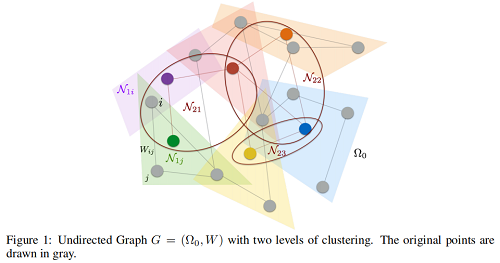可以看到 Figure 1 中，

• $k= 1$：
• 原始图有  $12$  个节点（即图上的  gray node），先分成  $6$  个  neighborhood $\mathcal{N}_{1 i}$ ，使用  $6$  个  feature maps  分别作用在不同的 $\mathcal{N}_{1 j}$  中，对每个  $\mathcal{N}_{1 j}$   进行   cluster  得到对应的  6  个聚类中心（with color）。
• $k=2$：
• 然后对上述产生的  $6$  个聚类中心，先分成  $3$  个neighborhood   $\mathcal{N}_{2 i}$，  再使用  $3$  个    feature maps  得到  $3$  个聚类中心。

图示只进行了  $2$  次。

## 2.3 Deep Locally Connected Networks

本文提出的空域架构也叫做深度局部连接网络 (Deep Locally Connected Networks) 。在这 个架构中使用了网络的多尺度聚类，事实上这里的尺度可以认为是下采样的层数，在这里我们考虑 $K$ 个尺度，实际上也就是说这个架构由 $K$ 个卷积层，每个卷积层后面都有一个池化层 (也就是进行一次聚类)，因此这个架构中总共有 $K$ 层，每层包括一个个卷积层和一个池化层。

我们设置   $\Omega_{0}=\Omega$   ，也就是输入层的节点集合（可以认为是第 $0$ 层），每一层的节点集合记作   $\Omega_{k}$ ，这里 $k=1,2, \cdots, K ， \Omega_{k}$  是利用   $\Omega_{k-1}$    的节点集合聚合成  $d_{k}$   个  $N_{k, i}$  的一个形式。另外定义    $\Omega_{k-1}$ 的节点的邻居节点集合的表示：

$\mathcal{N}_{k}=\left\{\mathcal{N}_{k, i} ; i=1 \ldots d_{k-1}\right\}$

PS:    $N_{k}$    的下标虽然为  $k$   ，但它代表的是第    $k-1$    的节点集合    $\Omega_{k-1}$    的每个节点的邻域的表示，里面的每个   $N_{k, i}$    都是一个集合。

根据上述定义，可以定义网络有 $k$ 层。

1. 假设  Input  是   $\Omega_{0}$   上的   real signal，以   $f_{k}$  来 代表第  $k$  层的卷积核的数量，即第 $k$ 层  feature map  的数量和信号的维度。
2. 每一层都会将   $\Omega_{k-1}$   上的  $f_{k-1}$  维的信号转换成  $\Omega_{k}$  上的  $f_{k}$  维的信号。（池化，切记）

结果：每一层的节点数量降低，但卷积核的数量会逐层增加使得特征的维度会变化。即：

①空间分辨率降低 (loses spatial resolution)，即每个$x_{k, i}$维度生变化，从 $d_{{k-1}}\times 1$ 到 $d_{{k}}\times 1$ ；
②滤波器数目增加 (increases the number of filters)，即  $F_{k, i, j}$  逐层增加。（缺点之一：卷积核不可共用）

第  $k$  层的输出  $x_{k+1}$  就被定义为:

$x_{k+1, j}=L_{k} h\left(\sum \limits _{i=1}^{f_{k-1}} F_{k, i, j} x_{k, i}\right) \quad\left(j=1 \ldots f_{k}\right)\quad \quad\quad\quad(2.1)$

其中：

• 第  $k$  层的输入节点用  $x_{k}=\left(x_{k, i} ; i=1,2, \cdots, f_{k-1}\right)$  来表示，大小是一个  $d_{k-1} \times f_{k-1}$  的矩阵；
• $x_{k, i}$  相当于第  $k$  层的第  $i$  个节点的特征。$x_{k, i}$ 的大小为 $d_{{k-1}}\times 1$；
• $F_{k, i, j}$  代表第  $k$  层的第  $j$  个卷积核对第  $k-1$  层的第  $i$  个 $x_{k-1, i}$ 进行卷积。$F_{k, i, j}$  是一个  $d_{k-1} \times d_{k-1}$  的稀疏矩阵，矩阵的第  $m$  行的非零值都只会存在于  $N_{k, m}$  所指定的第  $m$  个节点的邻居节点位置。
• $h$  代表非线性激活函数；
• $L_{k}$  代表对卷积的结果进行池化操作来降低节点的维度，$L_{k}$  相当于聚类的结果，是一个  $d_{k} \times d_{k-1}$  的稀疏矩阵，每一行指示一个簇的分布；
• 如果采用平均池化，那么  $L_{k}$ 的一个例子（  $d_{k}=3$, $d_{k-1}=6$  ) 可以是:

$L_{k}=\left|\begin{array}{cccccc}1 & 0 & 0 & 0 & 0 & 0 \\0 & \frac{1}{2} & 0 & \frac{1}{2} & 0 & 0 \\0 & 0 & \frac{1}{3} & 0 & \frac{1}{3} & \frac{1}{3}\end{array}\right|$

Eq 2.1 的优点：

这样就输出了聚集后新的节点特征，并且完成了降维的操作。同时，加入了一个可学习的卷积核。非常巧妙地将传统的 CNN 迁移到了图上，这就不再要求待处理数据具有平移不变性。完美地适应于图结构数据的节点无序、邻居个数不确定的特性。

Eq 2.1 的缺点：

首先,在更新中心 节点特征时利用上一层的节点特征不一定是该中心节点的邻居节点。谱域的 GCN 是基于拉普拉斯矩阵的，没有利用含有邻居学习的邻接矩阵，所以不具备局部性。其次，该方法的计算复杂度为 $O(n^{3})$ ，计算开销非常大，每次都需要对拉普拉斯矩阵进行特征分解。

整个过程可以用下图来表示：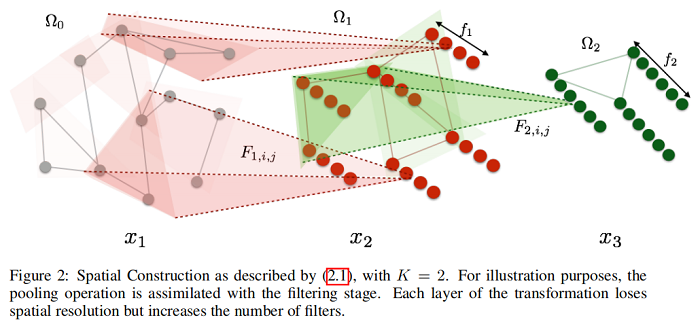通过以下过程构建第  $k$   层的  $W_{k}$   和  $N_{k}$ :

${\large \begin{array}{l}W_{0} &=W \\A_{k}(i, j) &=\sum \limits _{s \in \Omega_{k}(i)} \sum\limits_{t \in \Omega_{k}(j)} W_{k-1}(s, t),(k \leq K) \\W_{k} &=\text { rownormalize }\left(A_{k}\right),(k \leq K) \\\mathcal{N}_{k} &=\operatorname{supp}\left(W_{k}\right) \cdot(k \leq K)\end{array}}$

$A_{k}(i, j)$  的计算指：由于  $\Omega_{k}$  中的节点来自  $\Omega_{k-1}$  中的节点的聚类，所以  $i, j$  之间的权重是  $i$  和  $j$  对应的聚类之前的  $\Omega_{k-1}$  中节点之间所有权重的累加。

$\Omega_{k}$  是对  $\Omega_{k-1}$  的聚类点集合，聚类结果是若干个  $\mathcal{N}_{ki}$  。这里图聚类的方法采用  $W_{k}$  的  $\epsilon$  - covering，使用核函数  $K$  的  $\Omega$  的  $\epsilon$  - covering是一个划分  $P=\left\{P_{1}, P_{2}, \cdots, P_{n}\right\}$ ，满足：（可以参考本博客的谱聚类

$\underset{n}{sup} \quad \underset{x, x^{\prime} \in P_{n}}{sup} K\left(x, x^{\prime}\right) \geqslant \epsilon$

以   $S_{k}$  代表  $\mathcal{N}_{k}$  中的平均节点数量，那么第   $k$   层用来学习的参数量为:

$O\left(S_{k} \cdot\left|\Omega_{k}\right| \cdot f_{k} \cdot f_{k-1}\right)=O(n)$

实践中通常有   $S_{k} \cdot\left|\Omega_{k}\right| \approx \alpha \cdot\left|\Omega_{k-1}\right|$，$\alpha$   是下采样因子，满足  $\alpha \in(1,4)$。

这种架构的优点在于不需要很强的 regularity assumptions on the graph，只需要图具备低维邻域结构即可，甚至不需要很好的 global embedding。但是缺点在于没办法进行参数共享，对于每一层的每一个节点都要有单独的参数进行卷积而不能像CNN那样使用同一个卷积核在数据网格上进行平移。

# 3 Spectral Construction

The global structure of the graph can be exploited with the spectrum of its graph-Laplacian to generalize the convolution operator.

## 3.1 Harmonic Analysis on Weighted Graphs

### 3.1.1 Laplacian matrix

下面的内容有点水平的同学可以参考论文《Discrete Regularization》和《The Emerging Field of Signal Processing on Graphs

先介绍几种  Laplacian matrix：

① combinatorial Laplacian，组合拉普拉斯矩阵：

$L=D-W$

其中的元素为：

$L_{i, j}=\left\{\begin{array}{l}d_{i}\quad &i=j \\-w_{i j}\quad& i \neq j \text { and } v_{i} \text { is adjacent to } v_{j} \\0 \quad&\text { otherwise }\end{array}\right.$

② Symmetric normalized Laplacian，对称归一化的拉普拉斯矩阵：

$L^{s y s}=D^{-1 / 2} L D^{-1 / 2}=I-D^{-1 / 2} W D^{-1 / 2}$

其中的元素为：

$L_{i, j}^{s y s}=\left\{\begin{array}{l}1 \quad&i=j \text { and } d_{i} \neq 0 \\-\frac{w_{i j}}{\sqrt{d_{i} d_{j}}}\quad &i \neq j \text { and } v_{i} \text { is adjacent to } v_{j} \\0 \quad&\text { otherwise }\end{array}\right.$

③ Random walk normalized Laplacian，随机游走归一化拉普拉斯矩阵：

$L^{r w}=D^{-1} L=I-D^{-1} W$

其中的元素为：

$L_{i, j}^{r w}=\left\{\begin{array}{l}1\quad &i=j \text { and } d_{i} \neq 0 \\-\frac{w_{i j}}{d_{i}}\quad&i \neq j \text { and } v_{i} \text { is adjacent to } v_{j} \\0\quad &\text { otherwise }\end{array}\right.$

为方便，本文使用的是 ① combinatorial Laplacian 。

### 3.1.2 Introduction of smoothness function

对于一个固定的加权邻接矩阵  $W$  ，不同的节点信号列向量  $x \in \mathbb{R}^{m}$  (也就是说网络有  $m$  个节点) 有不同的平滑性 (smoothness) 度量  ，在节点  $i$  处的平滑性度量  $\|\nabla x\|_{W}^{2}(i)$ 为：

$\|\nabla x\|_{W}^{2}(i)=\sum \limits _{i} W_{i j}[x(i)-x(j)]^{2}$

所有  node  的平滑性度量为：

$\|\nabla x\|_{W}^{2}=\sum \limits _{i} \sum\limits_{j} w_{i j}[x(i)-x(j)]^{2}$

其实   $\|\nabla x\|_{W}^{2}$ 就是   $x^{T} L x$ ，可以参考《图神经网络基础二：谱图理论》
根据上面的公式可以得出最平滑的信号   $x$    ，对应于特征值为 $0$ 时，此刻的  $x$  其实是一个归一化（前文图神经网络没有归一化，这里归一化并不影响）的全 $1$ 的特征向量  $v_0$  为:

$v_{0}=\underset{x \in \mathbb{R}^{m} \ \|x\|=1}{\arg \min }\|\nabla _x\|_{W}^{2}=(1 / \sqrt{m}) 1_{m}$

事实上  $\mathbb{R}^{m}$  空间中最平滑的  $m$  个相互正交的单位向量其实就是   $L$  的特征向量（谱分解得出）:

$v_{i}=\underset{x \in \mathbb{R}^{m}\ \ \|x\|=1\ \ \ x \perp\left\{v_{0}, \ldots, v_{i-1}\right\}}{arg min} \|\nabla x\|_{W}^{2}$

每个 eigenvector $v_{i}$ 的平滑性度量的值其实就是特征值  $\lambda_{i}$注意  $x \text { in }\left[v_{0}, \ldots v_{m-1}\right]$

拉普拉斯矩阵的谱分解为 $L=V \Lambda V^{-1}=V \Lambda V^{T}$ ，其中  $\Lambda$  为特征值构成的对角矩阵， $V$  为特征向量构成的正交矩阵， $V$  的每一列都是一个特征向量，通过计算  $v_{i}^{T} L v_{i}$ 就可得到对应的特征值  $\lambda_{i}$ ，因此最平滑的信号向量就是特征值最小的特征向量，拉普拉斯矩阵的特征值就代表特征向量的平滑度。

上面提到的一组特征向量其实就是  $\mathbb{R}^{m}$   空间的一组基，前面的文章里说过图傅里叶变换其实就是将信号向量投影到拉普拉斯矩阵的各个特征向量上:

$F\left(\lambda_{k}\right)=\sum \limits _{i=1}^{m} x(i) v_{k}(i)$

特征值的大小表示平滑程度，它对应传统傅里叶变换中的频率，频率高表示短时间内变动多，和这里的相邻节点变动大（变动越大越不平滑）能对应起来。因此图傅里叶变换就是在将一个图信号分解到不同平滑程度的图信号上，就像传统傅里叶变换将函数分解到不同频率的函数上一样。

一个任意信号向量   $x$   分解到所有的特征向量上对应的每个系数用  $a_{i} \quad\left(a_{i}=F\left(\lambda_{i}\right)\right.$  表示 ，这些系数也就是图傅里叶变换后的系数) 表示，  $x$  可以表示为 $\sum \limits_{i=1}^{m} a_{i} v_{i}$  ，也就是  $V a$  ，那么  $x$  的平滑性度量的值可以用这些系数和特征值表示：

$\begin{array}{l}x^{T} L x&=(V a)^{T} L V a \\&=a^{T} V^{T} L V a \\&=a^{T} V^{T} V \Lambda V^{T} V a \\&=a^{T} I \Lambda I a \\&=a^{T} \Lambda a \\&=\sum \limits _{i=1}^{m} a_{i}^{2} \lambda_{i}\end{array}$

## 3.2 Extending Convolutions via the Laplacian Spectrum

### 3.2.1 Convolution Theorem

首先回顾一下卷积定理：

两个函数  $f$  和    $g$  进行卷积可以应用卷积定理，用公式表达卷积定理就是：

$F(f * g)=F(f) \cdot F(g)$

对于网络来说，直接进行卷积是困难的，因为网络不具备图像那样规则的网格结构，因此考虑应用图傅里叶变换将网络的空域信息映射到频域来应用卷积定理完成卷积操作。

应用卷积定理可以在频域上进行图的卷积操作，具体的方法是将滤波器  $h$  和图信号  $x$  都通过图傅里叶变换转换到频域然后计算转换后的结果的乘积（哈达玛积，也就是向量对应元素相乘），然后将相乘的结果再通过图傅里叶逆变换转换回空域，整个过程如下：

$\begin{array}{l}h * x&=F^{-1}\{F\{h\} \cdot F\{x\}\} \\&=F^{-1}\{\widehat{h} \cdot \widehat{x}\} \\&=F^{-1}\left\{\widehat{h} \cdot V^{T} x\right\} \\&=F^{-1}\left\{\operatorname{diag}\left[\widehat{h}_{1}, \cdots, \widehat{h}_{m}\right] V^{T} x\right\} \\&=\operatorname{Vdiag}\left[\widehat{h}_{1}, \cdots, \widehat{h}_{m}\right] V^{T} x\end{array}$

这里不妨定义  $V$  是graph Laplacian $L$ 中按特征值排序的特征向量  ，将   $\widehat{h}$   组织成对角矩阵  $\operatorname{diag}\left[\widehat{h}_{1}, \cdots, \widehat{h}_{m}\right], \widehat{h}_{1}, \cdots, \widehat{h}_{m}$  也就是神经网络要学习的参数。

### 3.2.2 Frequency Domain Architecture(频域架构)

在仍使用  $k$  来代表网络的第  $k$  层，  $k=1,2, \cdots, K ， f_{k}$   仍然代表卷积核的数量。该架构形同上述卷积定理，首先来描述卷积的过程，之后再描述如何进行下采样。因此现在假设第   $k$   层和第   $k+1$   层的节点数都是  $|\Omega|$ ，那么第   $k$  层的输入  $x_{k}$   就 是一个  $|\Omega| \times f_{k-1}$  的矩阵，输出  $x_{k+1}$  就是一个  $|\Omega| \times f_{k}$  的矩阵。第  $k$  层的计算过程可以 表示为:

$x_{k+1, j}=h\left(V \sum \limits_{i=1}^{f_{k-1}} F_{k, i, j} V^{T} x_{k, i}\right) \quad\left(j=1,2, \cdots, f_{k}\right)$

Where

• $x_{k, i}$  仍然相当于第  $k$ 层第   $i$  个输入信号；
• $F_{k, i, j}$   也就是第   $j$  个卷积核中对第  $k$ 层第    $i$   个输入信号进行卷积，每一个   $F_{k, i, j}$   都是一个对角矩阵，即   $\operatorname{diag}\left[\widehat{h}_{1}, \cdots, \widehat{h}_{m}\right]$  （For me：卷积核的组合矩阵）；
• $h$  仍然表示非线性激活；
• $V$   的每一列的特征向量是按照特征值的大小依次排列的 (降序)。

通常拉普拉斯矩阵只有前  $d$  个特征向量有意义，因为后面的特征向量对应的特征值比较小，特征向量非常的平滑，因此实际中可取拉普拉斯矩阵的前  $d$   列构成的矩阵  $V_{d}$  代替  $V$  ，这个过程就相当于频域架构的下采样的过程。这里的  $d$  相当于空域架构中的  $d_{k}$ (For me：降维) ，每 一层可以取不同的值。按照目前这种架构所需的参数复杂度为  $f_{k-1} \cdot f_{k} \cdot d=O(|\Omega|)$ 。

# 4 Numerical Experiments

本文中提出的两种架构在两个数据集上进行了实验验证效果。具体实验设置参看原论文，这里不做赘述。

## 4.1 Subsampled MNIST

这个数据集是从  MNIST  数据集的每张图片（  $28 \times 28$）上采样  $400$  个样本点构建图。实验样本如下图：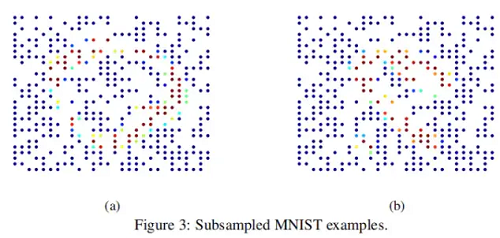实验结果如下图所示：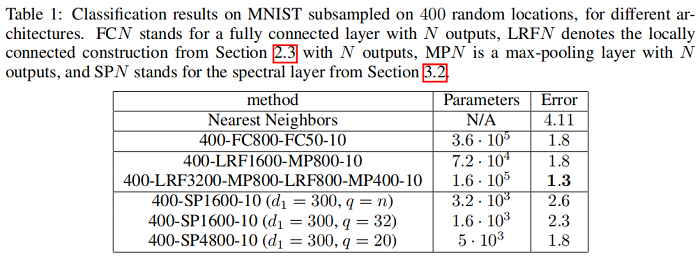## 4.2  MNIST on the sphere

这个数据集将MNIST数据集中的样本提升到3维空间中。实验样本如下图：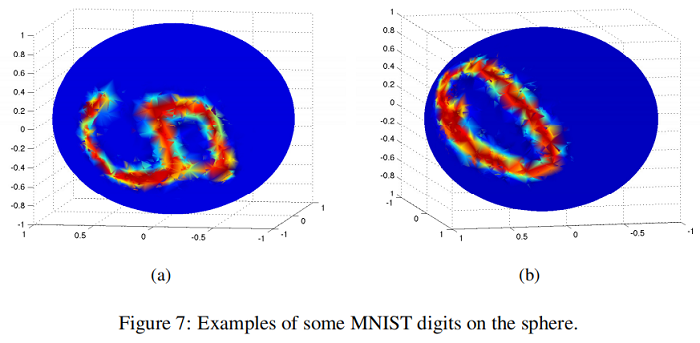实验结果如下图所示：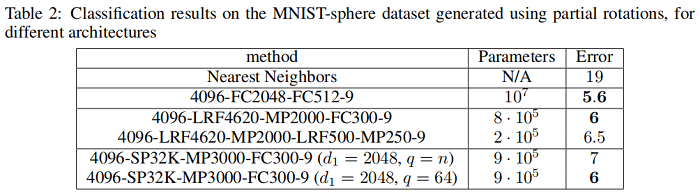# Reference

图神经网络基础目录：

Last modify ：2022-01-20  15:10:59

『总结不易，加个关注呗！』

posted @ 2022-01-17 00:54  Learner-  阅读(394)  评论(0编辑  收藏  举报